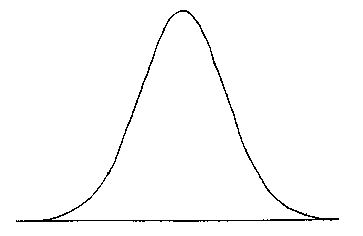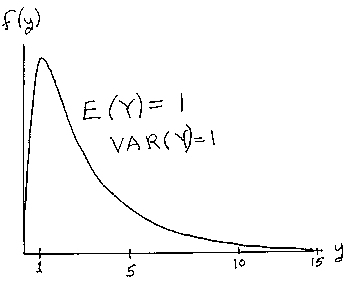LEGACY CONTENT. If you are looking for Voteview.com, PLEASE CLICK HERE

This site is an archived version of Voteview.com archived from University of Georgia on May 23, 2017. This point-in-time capture includes all files publicly linked on Voteview.com at that time. We provide access to this content as a service to ensure that past users of Voteview.com have access to historical files. This content will remain online until at least January 1st, 2018. UCLA provides no warranty or guarantee of access to these files.

### 45-733 PROBABILITY AND STATISTICS I

The Normal or Gaussian Distribution

The Normal or Gaussian Distribution is written as:

f(x) = {1/[(2p)1/2 s]} e-(x - m)2/ 2s2)              -¥ < x < +¥

Where E(X) = m    and VAR(X) = s2The log-normal distribution is the distribution of a random variable whose natural logarithm follows a normal distribution. This distribution is used in the Natural Sciences to model inherently postive phenomenon such as size -- for example, by volume or weight. The growth of bacteria colonies and of individual animals can be usefully analyzed using the log-normal distribution.

If X ~ N(m, s2), then Y = eX ~ log-normal. Namely:

f(y) = {1/[(2p)1/2 ys]} e-(lny - m)2/ 2s2)

Note that m and s2 are the mean and variance of X. The mean of Y is:

E(Y) = e[m + s2/2]The Normal Distribution was discovered by Carl Friedrich Gauss (1777-1855). Gauss was probably the greatest genius of whom there is any historical record. Gauss could do arithmetic at the age of 3 and fortunately for the history of humanity Gauss's unusual abilities drew the attention of the Duke of Brunswick (northern Germany) who financed Gauss's education.

At the age of 14 or 15 Gauss discovered the Prime Number Theorem later proved by Riemann in 1896 (the Prime Number Theorem is concerned with the average distribution of the Prime Numbers). About this time Gauss also invented The Principle of Least Squares which is the basis for all modern estimation methods. Just this creation alone was a towering intellectual achievement of the first order given its pervasive use throughout the Sciences.

Also about this time Gauss discovered (or created!) the Normal Distribution in the theory of probability.

At the age of 18 Gauss proved a beautiful theorem about the constructability of regular polygons by a ruler and compass -- a problem that had been worked on since the Ancient Greeks. Gauss not only discovered a new constructable Polygon -- 17 sided, the first in 2000 years -- he also proved a general theorem about just what Polygons were constructable!

At the age of 21 in 1798, Gauss proved The Fundamental Theorem of Arithmetic (every integer greater than 1 can be expressed as a product of prime numbers). One year later in 1799, Gauss proved The Fundamental Theorem of Algebra (every polynomial equation with real or complex coefficients has a set of real or complex roots). Gauss's method of mathematical proof that he used in these two magnificent achievements marked the advent of the modern rigorous approach to mathematics. Gauss's proofs were terse, thorough, and rigorous, and his approach set the standard followed by later mathematicians.

About 1805 Gauss was asked to solve an important astronomical problem -- determining the orbit of an asteroid named Ceres. Ceres was difficult to see with telescopes in use at that time and only fragmentary observations of its orbit existed. Georg Wilhelm Hegel wrote a remarkable tract about this time criticizing astronomers for not consulting philosophers. Hegel believed that philosophy could "prove" that no further planets could exist so there was no need to look for Ceres! (Hegel's later work was used by Marx!) Gauss solved the problem using his method of least squares (note that he fitted an elipse to the fragmentary data) and his unparalleled ability at numerical computation. He forecast exactly where Ceres would appear when it came from behind the sun and indeed he was exactly right. This achievement caused a sensation in Europe comparable to the confirmation of Einstein's famous theory of how gravity should bend light.

Sometime in the 1820s Gauss developed all the main results in Non-Euclidean Geometry. These were not known until after his death. About the same time he developed The Intrinsic Differential Geometry of General Curved Surfaces. These results allow the determination of geodesic curves. These ideas were so powerful that they enabled the development of Riemannian geometry, tensor analysis, and the ideas of Einstein.

In the early 1830s Gauss developed modern Complex Numbers ( a + ib). This and his other work on numbers lead directly to modern algebraic number theory.

The above is only a fraction of Gauss's achievements. He also did fundamental work on the theory of magnetism, the theory of functions of a complex variable, and the theory of elliptic functions, just to list a few areas. Much of the "wallpaper" of our advanced civilization can be traced back to this colossal genius. As one author puts it: "He surpassed the levels of achievement possible for ordinary men of genius in so many ways that one sometimes has the eerie feeling that he belonged to a higher species."

For more information on Gauss and other mathematicians, see the University of St. Andrews website:

St. Andrews Website